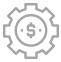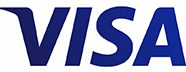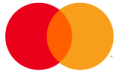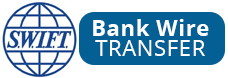# The principles behind lots trading and pips calculation

## What you will learn:

• Lot definition
• Different Lot sizes explained
• USD and EUR practical illustrations
• The correlation between margin and leverage
• Understanding the intrigues in Margin Call calculation

## What is a Lot Size in Forex?

In Forex trading, a standard Lot refers to a standard size of a specific financial instrument. It is one of the prerequisites to get familiar with for Forex starters.

## Standard Lots

This is the standard size of one Lot which is 100,000 units. Units referred to the base currency being traded. When someone trades EUR/USD, the base currency is the EUR and therefore, 1 Lot or 100,000 units worth 100,000 EURs.

## Mini Lots

Now, let’s use smaller sizes. Traders use Mini Lots when they wish to trade smaller sizes. For example, a trader may wish to trade only 10,000 units. So when a trader places a trade of 0.10 Lots or 10,000 base units on GBP/USD, this means that he trades 10,000 British Pounds.

## Micro Lots

There are many beginners or small investors who wish to use the smallest possible Lots sizes. In contrary to the Mini Lots that refer to 10,000 units, traders are welcome to trade 1,000 units or 0.01. For example, when someone trades USD/CHF with a Micro Lot the trader basically trades 1,000 USDs.

## Pip Value

Now that we understand what Lots are, let’s take one step further. We need to calculate the Pip Value so we can estimate our profits or losses from our trading.

The simplest way to calculate the Pip Value is to first use the Standard Lots. You will then have to adjust your calculations so you can find the Pip Value on Mini Lots, Micro Lots or any other Lot size you wish to trade.

## USD Base Currency

Our calculations in this sector are when your Base currency is the USD. We will provide three different examples.

USD quote currency of the currency pair. You’re trading 1 standard Lot (100,000 base units) that the quote currency is the USD such as EUR/USD. The Pip Value is calculated as below:

#### 100,000*0.0001 (4th decimal)=\$10

USD base currency of the currency pair. You’re trading 1 standard Lot (100,000 base units) and the base currency is the USD such as USD/JPY. The Pip Value is calculated as below:

The USD/JPY is traded at 99.735 means that \$1=99.73 JPY 100,000*0.01 (the 2nd decimal) /99.735≈\$10.03. We approximated because the exchange rate changes, so does the value of each pip.

Finding the Pip Value in a currency pair that the USD is not traded. You’re trading 1 standard Lot (100,000 base units) on GBP/JPY.

The GBP/JPY is traded at 153.320. Because the value changes in the quote currency times the exchange rate ratio as

#### The Pip Value => 100,000*0.01JPY*1GBP/153.320JPY = 6.5 GBP

Because the base currency of the account is the USD then we need to take into account the GBP/USD rate which let’s assume that is currently at 1.53560.

## EUR Base Currency

Now let’s make our examples when the Base Currency of our account is the EUR

EUR base currency of the currency pair. You’re trading 1 standard Lot (100,000 base units) on EUR/USD. The Pip Value is calculated as below

The EUR/USD is traded at 1.30610 means that 1 EUR=\$1.30 USD so

#### 100,000*0.0001 (4th decimal)/1.30610 ≈7.66 EUR

Finding the Pip Value in a currency pair that the EUR is not traded. You’re trading 1 standard Lot (100,000 base units) on GBP/JPY. From our example before, we know that the value is 6.5 GBP. Now, we need to take into account the EUR/GBP rate in order to calculate the Pip Value. Let’s assume that the rate is currently at 0.85000. So:

## Leverage – How it works

You are probably wondering how can I trade with Lot sizes of 100,000 base units or even 1,000 base units. Well, the answer is very simple. This is available to you from the leverage you have in your account. So let’s assume that your account’s leverage is set at 100:1. This means that for every \$1 used, you’re actually trading \$100 in the Forex market. In order for you to trade a position of \$100,000 then the required margin to open such a position will be \$1,000. As for any losses or gains these will be deducted or added to the remaining balance in your account.

If your account’s leverage is set at 200:1 this means that for every \$1 you use you’re actually trading \$200. So for a trade of \$100,000 you will require a margin to be at \$500.

## Margin Call – What you should know

Now looking at the examples above regarding the leverage you’re probably thinking that is the best to work with the highest possible leverage. However, you need to take into consideration your Margin requirements as well as the risks associated with higher leverages.

Let’s just say that you have deposited first \$5,000 to your trading account that the leverage is set at 100:1. Your nominated currency is the USD. The first time you will login to your MT4 trading account you will notice that the Balance and the Equity is \$5,000 and this is due to the fact that you did not place any trades yet.

Now, you have decided to open a position on the USD/CHF of the 1 standard Lot which means that you will require use a margin of \$1,000. The floating P/L is at -9.55. The account will show the following

Balance Equity Margin Free Margin Margin Level
5,000 4,990.45 (5,000-9.55) 1,000 3,990.45 (4,990.45-1000) 499.05% (4990.45/1000)*100

If your Forex Broker Margin Call level is set at 100% this means that when the Margin Level reaches this percentage it will notify you to add more funds. As you can understand from the example above, the P/L, and your Margin will affect your Margin Level. Now, if your Broker sets the Stop Out Level at 50% this means that your position will be closed by the Broker when the Margin Level reaches that level.

Let’s use another example when your leverage is set at 200:1. We will use the same example above to understand how the leverage will affect your Margin Level. Your account will show the following

Balance Equity Margin Free Margin Margin Level
5,000 4,990.45 500 4,490.45 998.10%

By looking at the numbers above, you will prefer to use a higher leverage for your account. However, let’s assume that the market goes against you and you have bought 9 Lots of USD/CHF but the pair falls. When you open your position you will have the following numbers:

Balance Equity Margin Free Margin Margin Level
5,000 4,990.45 4,500 (900,000/200) 490.45 110.90%

As we explained above, the broker will give you a Margin Call when you have 100% margin level. This means that you will receive a Margin Call when the USD/CHF falls 5 pips only. On the other hand, if you had a Leverage set at 100:1 the would not allow you to enter into such a position from the first place and you would have saved your equity.

## That's the end of the Beginner Course,lets move on to another learning section

###Money Management

•••••